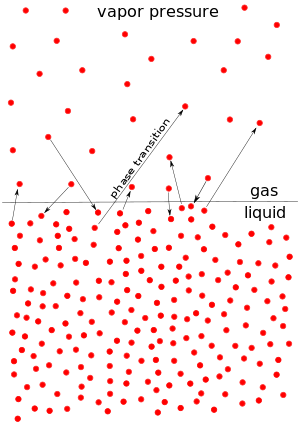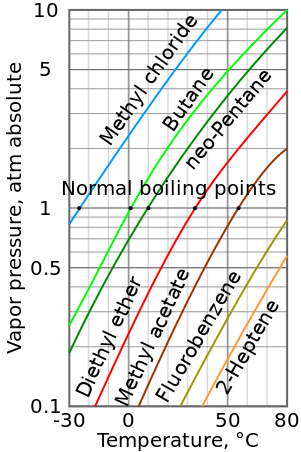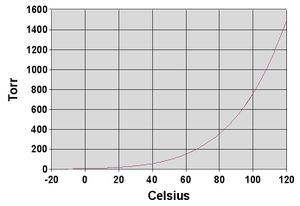# ضغط البخار

(تم التحويل من ضغط بخار)The microscopic process of evaporation and condensation at the liquid surface.
The "Pistol Test Tube" experiment. The tube contains alcohol and is closed with a piece of cork. By heating the alcohol, the vapors fill in the space, increasing the pressure in the tube to the point of the cork popping out. (Warning: this experiment should only be performed with safety glasses on!)

ضغط البخار هو ضغط البخار في حالة التعادل الترموديناميكي مع أطوار المادة الأخرى اللابخارية. جميع المواد السائلة والصلبة تميل إلى التبخر إلى الحالة الغازية وجميع الغازات تميل إلى التكاثف لتعود إلى حالتها الأصلية (صلبة كانت أم سائلة). وعند أي درجة حرارة لمادة معينة، هناك ضغط يكون عنده غاز هذه المادة في حالة تعادل ديناميكي مع أطواره السائلة أو الصلبة. وهذا ما يسمى بضغط البخار لهذه المادة عند درجة الحرارة هذه.

يشير ضغط بخار مادة إلى معدل تبخر السائل، ويتعلق بميل الجزيئات والذرات إلى الهرب من السائل أو الصلب والتحول إلى الحالة الغازية.

The vapor pressure of any substance increases non-linearly with temperature according to the Clausius–Clapeyron relation. The atmospheric pressure boiling point of a liquid (also known as the normal boiling point) is the temperature at which the vapor pressure equals the ambient atmospheric pressure. With any incremental increase in that temperature, the vapor pressure becomes sufficient to overcome atmospheric pressure and lift the liquid to form vapor bubbles inside the bulk of the substance. Bubble formation deeper in the liquid requires a higher temperature due to the higher fluid pressure, because fluid pressure increases above the atmospheric pressure as the depth increases. More important at shallow depths is the higher temperature required to start bubble formation. The surface tension of the bubble wall leads to an overpressure in the very small, initial bubbles.

The vapor pressure that a single component in a mixture contributes to the total pressure in the system is called partial pressure. For example, air at sea level, and saturated with water vapor at 20 °C, has partial pressures of about 2.3 kPa of water, 78 kPa of nitrogen, 21 kPa of oxygen and 0.9 kPa of argon, totaling 102.2 kPa, making the basis for standard atmospheric pressure.

. . . . . . . . . . . . . . . . . . . . . . . . . . . . . . . . . . . . . . . . . . . . . . . . . . . . . . . . . . . . . . . . . . . . . . . . . . . . . . . . . . . . . . . . . . . . . . . . . . . . . . . . . . . . . . . . . . . . . . . . . . . . . . . . . . . . . . . . . . . . . . . . . . . . . . . . . . . . . . . . . . . . . . . .

## القياس والوحدات

Vapor pressure is measured in the standard units of pressure. The International System of Units (SI) recognizes pressure as a derived unit with the dimension of force per area and designates the pascal (Pa) as its standard unit. One pascal is one newton per square meter (N·m−2 or kg·m−1·s−2).

Experimental measurement of vapor pressure is a simple procedure for common pressures between 1 and 200 kPa. Most accurate results are obtained near the boiling point of substances and large errors result for measurements smaller than 1kPa. Procedures often consist of purifying the test substance, isolating it in a container, evacuating any foreign gas, then measuring the equilibrium pressure of the gaseous phase of the substance in the container at different temperatures. Better accuracy is achieved when care is taken to ensure that the entire substance and its vapor are at the prescribed temperature. This is often done, as with the use of an isoteniscope, by submerging the containment area in a liquid bath.

Very low vapor pressures of solids can be measured using the Knudsen effusion cell method.

In a medical context, vapor pressure is sometimes expressed in other units, specifically millimeters of mercury (mmHg). This is important for volatile anesthetics, most of which are liquids at body temperature, but with a relatively high vapor pressure.

## تقدير ضغوط البخار بمعادلة أنطوان

معادلة أنطوان is a pragmatic mathematical expression of the relation between the vapor pressure and the temperature of pure liquid or solid substances. It is obtained by curve-fitting and is adapted to the fact that vapor pressure is usually increasing and concave as a function of temperature. The basic form of the equation is:

$\log P=A-{\frac {B}{C+T}}$and it can be transformed into this temperature-explicit form:

$T={\frac {B}{A-\log P}}-C$where:

$P$is the absolute vapor pressure of a substance
$T$is the temperature of the substance
$A$, $B$and $C$are substance-specific coefficients (i.e., constants or parameters)
$\log$is typically either $\log _{10}$or $\log _{e}$A simpler form of the equation with only two coefficients is sometimes used:

$\log P=A-{\frac {B}{T}}$which can be transformed to:

$T={\frac {B}{A-\log P}}$Sublimations and vaporizations of the same substance have separate sets of Antoine coefficients, as do components in mixtures. Each parameter set for a specific compound is only applicable over a specified temperature range. Generally, temperature ranges are chosen to maintain the equation's accuracy of a few up to 8–10 percent. For many volatile substances, several different sets of parameters are available and used for different temperature ranges. The Antoine equation has poor accuracy with any single parameter set when used from a compound's melting point to its critical temperature. Accuracy is also usually poor when vapor pressure is under 10 Torr because of the limitations of the apparatus[بحاجة لمصدر] used to establish the Antoine parameter values.

The Wagner equation gives "one of the best" fits to experimental data but is quite complex. It expresses reduced vapor pressure as a function of reduced temperature.

## العلاقة بنقاط غليان السوائلجدول لوغارثمي-خطي لضغط بخار لمختلف السوائل

As a general trend, vapor pressures of liquids at ambient temperatures increase with decreasing boiling points. This is illustrated in the vapor pressure chart (see right) that shows graphs of the vapor pressures versus temperatures for a variety of liquids. At the normal boiling point of a liquid, the vapor pressure is equal to the standard atmospheric pressure defined as 1 atmosphere, 760 Torr, 101.325 kPa, or 14.69595 psi.

For example, at any given temperature, methyl chloride has the highest vapor pressure of any of the liquids in the chart. It also has the lowest normal boiling point (−24.2 °C), which is where the vapor pressure curve of methyl chloride (the blue line) intersects the horizontal pressure line of one atmosphere (atm) of absolute vapor pressure.

Although the relation between vapor pressure and temperature is non-linear, the chart uses a logarithmic vertical axis to produce slightly curved lines, so one chart can graph many liquids. A nearly straight line is obtained when the logarithm of the vapor pressure is plotted against 1/(T + 230) where T is the temperature in degrees Celsius. The vapor pressure of a liquid at its boiling point equals the pressure of its surrounding environment.

## مخاليط السوائل

Raoult's law gives an approximation to the vapor pressure of mixtures of liquids. It states that the activity (pressure or fugacity) of a single-phase mixture is equal to the mole-fraction-weighted sum of the components' vapor pressures:

$P_{\rm {tot}}=\sum _{i}Py_{i}=\sum _{i}P_{i}^{\rm {sat}}x_{i}\,$where $P_{\rm {tot}}$is the mixture's vapor pressure, $x_{i}$is the mole fraction of component $i$in the liquid phase and $y_{i}$is the mole fraction of component $i$in the vapor phase respectively. $P_{i}^{\rm {sat}}$is the vapor pressure of component $i$. Raoult's law is applicable only to non-electrolytes (uncharged species); it is most appropriate for non-polar molecules with only weak intermolecular attractions (such as London forces).

Systems that have vapor pressures higher than indicated by the above formula are said to have positive deviations. Such a deviation suggests weaker intermolecular attraction than in the pure components, so that the molecules can be thought of as being "held in" the liquid phase less strongly than in the pure liquid. An example is the azeotrope of approximately 95% ethanol and water. Because the azeotrope's vapor pressure is higher than predicted by Raoult's law, it boils at a temperature below that of either pure component.

There are also systems with negative deviations that have vapor pressures that are lower than expected. Such a deviation is evidence for stronger intermolecular attraction between the constituents of the mixture than exists in the pure components. Thus, the molecules are "held in" the liquid more strongly when a second molecule is present. An example is a mixture of trichloromethane (chloroform) and 2-propanone (acetone), which boils above the boiling point of either pure component.

The negative and positive deviations can be used to determine thermodynamic activity coefficients of the components of mixtures.

. . . . . . . . . . . . . . . . . . . . . . . . . . . . . . . . . . . . . . . . . . . . . . . . . . . . . . . . . . . . . . . . . . . . . . . . . . . . . . . . . . . . . . . . . . . . . . . . . . . . . . . . . . . . . . . . . . . . . . . . . . . . . . . . . . . . . . . . . . . . . . . . . . . . . . . . . . . . . . . . . . . . . . . .

## المواد الصلبة

Equilibrium vapor pressure can be defined as the pressure reached when a condensed phase is in equilibrium with its own vapor. In the case of an equilibrium solid, such as a crystal, this can be defined as the pressure when the rate of sublimation of a solid matches the rate of deposition of its vapor phase. For most solids this pressure is very low, but some notable exceptions are naphthalene, dry ice (the vapor pressure of dry ice is 5.73 MPa (831 psi, 56.5 atm) at 20 °C, which causes most sealed containers to rupture), and ice. All solid materials have a vapor pressure. However, due to their often extremely low values, measurement can be rather difficult. Typical techniques include the use of thermogravimetry and gas transpiration.

There are a number of methods for calculating the sublimation pressure (i.e., the vapor pressure) of a solid. One method is to estimate the sublimation pressure from extrapolated liquid vapor pressures (of the supercooled liquid), if the heat of fusion is known, by using this particular form of the Clausius–Clapeyron relation:

$\ln \,P_{\rm {s}}^{\rm {sub}}=\ln \,P_{\rm {l}}^{\rm {sub}}-{\frac {\Delta _{\rm {fus}}H}{R}}\left({\frac {1}{T_{\rm {sub}}}}-{\frac {1}{T_{\rm {fus}}}}\right)$حيث:

• $P_{\rm {s}}^{\rm {sub}}$is the sublimation pressure of the solid component at the temperature $T_{\rm {sub}}.
• $P_{\rm {l}}^{\rm {sub}}$is the extrapolated vapor pressure of the liquid component at the temperature $T_{\rm {sub}}.
• $\Delta _{\rm {fus}}H$is the heat of fusion.
• $R$is the gas constant.
• $T_{\rm {sub}}$is the sublimation temperature.
• $T_{\rm {fus}}$is the melting point temperature.

This method assumes that the heat of fusion is temperature-independent, ignores additional transition temperatures between different solid phases, and it gives a fair estimation for temperatures not too far from the melting point. It also shows that the sublimation pressure is lower than the extrapolated liquid vapor pressure (ΔfusH > 0) and the difference grows with increased distance from the melting point.

## نقطة غليان الماءGraph of water vapor pressure versus temperature. Note that at the normal boiling point of 100°C, the vapor pressure equals the standard atmospheric pressure of 760 Torr or 101.325 kPa.

الماء، مثل كل السوائل، يبدأ في الغليان عندما يصل ضغط بخاره إلى مقدار الضغط المحيط. وفي الارتفاعات الشاهقة، فإن الضغط الجوي يكون أقل مما يجعل الماء يغلي عند درجة حرارة أقل. The boiling temperature of water for pressures around atmospheric pressure can be approximated by this Antoine equation:

$\log _{10}P=8.07131-{\frac {1730.63}{233.426+T_{b}}}$or transformed into this temperature-explicit form:

$T_{b}={\frac {1730.63}{8.07131-\log _{10}P}}-233.426$where the temperature $T_{b}$is the boiling point temperature in degrees Celsius and the pressure $P_{}$is in Torr.

## قاعدة دورنگ

Dühring's rule states that a linear relationship exists between the temperatures at which two solutions exert the same vapor pressure.

## أمثلة

The following table is a list of a variety of substances ordered by increasing vapor pressure (بوحدات مطلقة).

المادة ضغط البخار درجة الحرارة
(°C)
(Pa) (bar) (mmHg)
تنگستن 100 Pa 0.001 0.75 3203
Ethylene glycol 500 Pa 0.005 3.75 20
Xenon difluoride 600 Pa 0.006 4.50 25
ماء (H2O) 2.3 kPa 0.023 17.5 20
پروپانول 2.4 kPa 0.024 18.0 20
Methyl isobutyl ketone 2.66 kPa 0.0266 19.95 25
إثانول 5.83 kPa 0.0583 43.7 20
فريون 113 37.9 kPa 0.379 284 20
Acetaldehyde 98.7 kPa 0.987 740 20
Butane 220 kPa 2.2 1650 20
Formaldehyde 435.7 kPa 4.357 3268 20
Propane 997.8 kPa 9.978 7584 26.85
كبريتيد الكربونيل 1.255 MPa 12.55 9412 25
أكسيد النيتروز 5.660 MPa 56.60 42453 25
ثاني أكسيد الكربون 5.7 MPa 57 42753 20

. . . . . . . . . . . . . . . . . . . . . . . . . . . . . . . . . . . . . . . . . . . . . . . . . . . . . . . . . . . . . . . . . . . . . . . . . . . . . . . . . . . . . . . . . . . . . . . . . . . . . . . . . . . . . . . . . . . . . . . . . . . . . . . . . . . . . . . . . . . . . . . . . . . . . . . . . . . . . . . . . . . . . . . .

## المصادر

1. ^ Růžička, K.; Fulem, M. & Růžička, V. "Vapor Pressure of Organic Compounds. Measurement and Correlation" (PDF). Archived from the original (PDF) on 2010-12-26. Retrieved 2009-10-18.
2. ^ أ ب What is the Antoine Equation? (Chemistry Department, Frostburg State University, Maryland)
3. ^ أ ب Sinnot, R.K. (2005). Chemical Engineering Design] (4th ed.). Butterworth-Heinemann. p. 331. ISBN 978-0-7506-6538-4.
4. ^ Wagner, W. (1973), "New vapour pressure measurements for argon and nitrogen and a new method for establishing rational vapour pressure equations", Cryogenics 13 (8): 470–482, doi:10.1016/0011-2275(73)90003-9, Bibcode1973Cryo...13..470W
5. ^ Perry's Chemical Engineers' Handbook, 7th Ed. pp. 4–15
6. ^ Perry, R.H.; Green, D.W., eds. (1997). Perry's Chemical Engineers' Handbook (7th ed.). McGraw-Hill. ISBN 978-0-07-049841-9.
7. ^ Petrucci, Ralph H.; Harwood, William S.; Herring, F.Geoffrey (2002). General Chemistry (8th ed.). Prentice Hall. p. 484. ISBN 978-0-13-014329-7.
8. ^ Dreisbach, R. R. & Spencer, R. S. (1949). "Infinite Points of Cox Chart Families and dt/dP Values at any Pressure". Industrial and Engineering Chemistry. 41 (1). p. 176. doi:10.1021/ie50469a040.
9. ^ Moller B.; Rarey J.; Ramjugernath D. (2008). "Estimation of the vapour pressure of non-electrolyte organic compounds via group contributions and group interactions". Journal of Molecular Liquids. 143: 52–63. doi:10.1016/j.molliq.2008.04.020.
10. ^ "Thermophysical Properties Of Fluids II – Methane, Ethane, Propane, Isobutane, And Normal Butane" (page 110 of PDF, page 686 of original document), BA Younglove and JF Ely.
11. ^ "Thermophysical Properties Of Nitrous Oxide" (page 14 of PDF, page 10 of original document), ESDU.